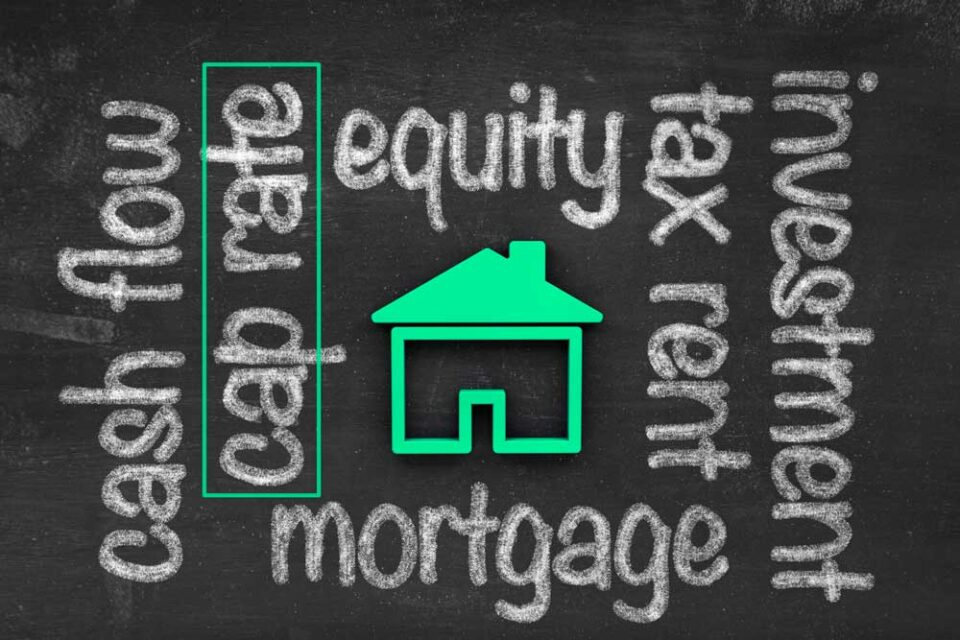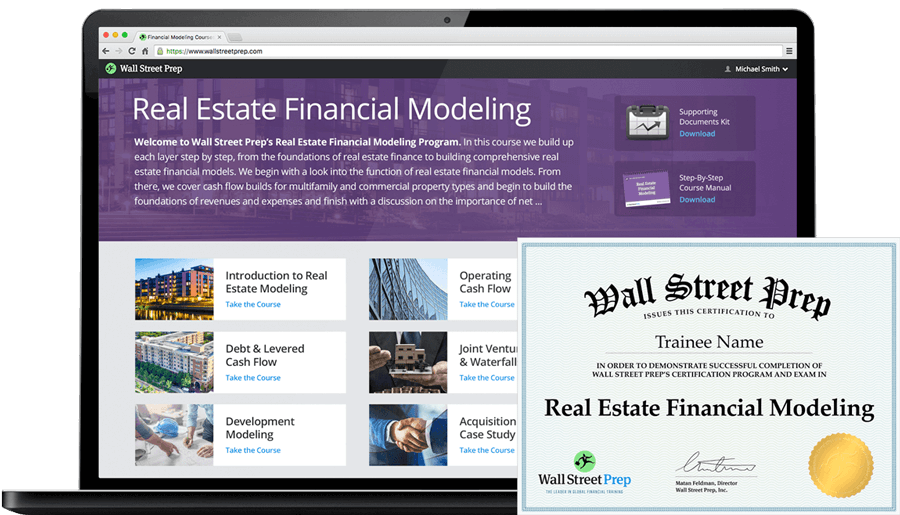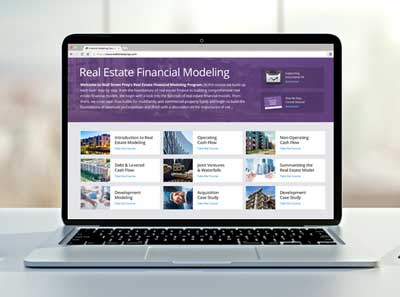# Cap Rate

Understand the Cap Rate Metric in Real Estate Investing## Cap Rate Formula

The formula for calculating the capitalization rate in real estate is as follows.

Cap Rate = Net Operating Income (NOI) / Property Value

For example, if a \$1 million investment property is generating \$50,000 in annual net operating income (rental fees less operating expenses), the cap rate on the investment is 5.0%.

• Cap Rate = \$50,000/ \$1 million = 5.0%

## How to Calculate Cap Rate: Property Example

A property with an asking price of \$1m and NOI of \$125k will have a 12.5% cap rate.

• Cap Rate = \$125k / \$1m = 12.5%

If you’re specifically familiar with EV/ EBITDA multiples, the closest thing to a cap rate is an inverse EBITDA multiple because NOI as a measure of profit is closest to EBITDA (with some major differences which we address here).

Cap rates are the primary shorthand by which different real estate properties are compared by investors.

For example, if you’re evaluating a property with a \$10 million asking price and an expected NOI of \$1 million, that property would have a 10% cap rate.

All else equal, the higher the cap rate, the higher the annual return on investment.

However, a higher cap rate isn’t always better. For example, higher cap rates are often achieved by investing in riskier properties, so there is a tradeoff between risk and return.

## Observed Property Value and Cap Rates

To decide whether the asking price is appropriate, you’d look at the cap rates on similar properties.

If the cap rates of comparable properties are on average lower – say 8% – you might perceive the asking price as reasonable because it provides a higher NOI relative to the property value.

If you’re more comfortable thinking about multiples, simply invert the cap rate – in our example, you’d be comparing an asset valued at 10x (value/NOI) against a seemingly more expensive peer group trading at 12.5x (value/NOI).

Alternatively, cap rates are often used to help price properties.

For example, if an investor owns a property generating NOI of \$2 million but comparable properties trade at only 6% cap rates (perhaps due to higher risks with these types of properties), the investor would use the peer group cap rates to guide the investor’s pricing decision.

So at the 6% cap rate, the property would be priced at \$33.3 million.

• Property Value = \$2 million / 6% = \$33.3 million.

## Which Factors Determine the Cap Rate?

Just as with traditional multiples, there are many variables that can distort the comparison of properties using this metric, including:

1. Timing of NOI (LTM or forward)
2. Growth rates
3. Returns on capital
4. Cost of capital of properties (or REITs) being compared

However, in real estate, it is much easier to find comparable properties (with therefore similar growth, returns, and cost of capital profiles), which mutes the problems described above20+ Hours of Online Video Training

### Master Real Estate Financial Modeling

This program breaks down everything you need to build and interpret real estate finance models. Used at the world's leading real estate private equity firms and academic institutions.Inline FeedbacksLearn Real Estate Financial Modeling

Online Course: Everything you need to build and interpret real estate finance models.

X

The Wall Street Prep Quicklesson Series

7 Free Financial Modeling Lessons

Get instant access to video lessons taught by experienced investment bankers. Learn financial statement modeling, DCF, M&A, LBO, Comps and Excel shortcuts.